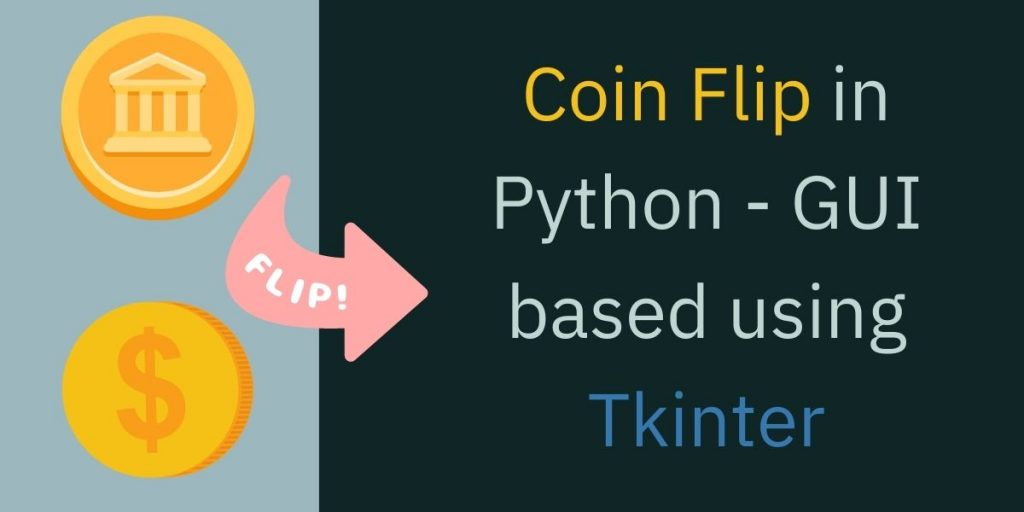# Coin Flip GUI using Python TkinterIn this tutorial, we’ll code a coin flip program with Graphical User Interface (GUI) using Python Tkinter. The tutorial is aimed at teaching you the basics of the Tkinter module, a great module for developing GUI-based programs in Python.

Also read: Tkinter Tutorial – Using Tkinter Buttons

## Implementing the Coin Flip GUI App in Python Tkinter

Tkinter is the standard GUI library for python which is used to make interface-based applications. Tkinter when combined with Python makes it very easy to make GUI-based applications.

### 1. Installing Modules

For this program, we need Python NumPy, Pillow, and Tkinter libraries which we can easily download using pip.

```pip install numpy
pip install pillow
pip install tk
```

### 2. Importing Modules

After installing the modules we can start coding our program by importing all modules in our program.

```import numpy as np
from tkinter import *
from PIL import Image, ImageTk
```

Note:- In the above code, ‘*’ means that we’re importing everything from module Tkinter.

### 3. Creating the Main Window for our Application

First, we’ll initialize the Tkinter class using Tk() object and assign it to the ‘root’ variable. So now by using root we can access all the methods of the Tkinter module.

In the second line of code, we specify the Widow Size of the output GUI screen, like here we’ve given it a value of 400*400(for widthxheight).

Now we use mainloop method to make the window persistent meaning that the window will not close unless we want to close it ourselves. If we skip this line of code, the output screen appears once and closes immediately.

```root = Tk()
root.geometry("500*500")
root.mainloop()
```

After running the above code we’ll get a window like shown below. If you get a window like this you are all good and can follow on.

We’ll show the image of a coin according to the outcome of our program. If the output is Heads then it will show the head side of the coin, and Tails side of the coin when it is Tails.

Heads and Tails both images are saved in the same directory as our program file. If not, in your case then you need to pass the image file name with the location of it.

```#load heads image

```

For your convenience, we have added the image file to the head and tail side of a coin.

Now that our main window is ready we need a button which we can press to Toss the coin.

By pressing this button we are just calling a tossTheCoin function. We can use the Button class in tkinter to create the button.

```b1=Button(root, text="Toss the Coin", font=("Arial", 10), command=tossTheCoin, bg='teal', fg='white', activebackground="lightblue", padx=10, pady=10)
b1.pack()
```

The above code will render a button in our main window with the text Toss the Coin. In command, we’ll pass our function name.

Note: We use the pack() method for every element we want to render on our main window.

### 6. Text Field for result

Now, we make a text field for our result of the coin flip in the text format. We do so, by using the code:

```tfield = Text(root, width=52, height=5)
tfield.pack()
```

To insert text in this text field we use the insert function, as follows:

```tfield.insert(INSERT, "Click on the Button.. To Flip the Coin and get the result")
```

Now, for every time change in the result, we will need to erase the previous inserted text, so we bind the delete text method with the button click –

```tfield.delete("1.0", "end")
```

### 7. Implementing tossTheCoin() function

When we toss a coin, it has a 50% chance that it lands on either head or tail. We want to have this fair nature in our program so it can be close to the real coin-tossing scenario. For this, we will use the binomial method of the NumPy module.

```np.random.binomial(1,0.5)
```

This will return either one or zero. So we can write if condition statement to check if it is 1 or 0 and render the head or tail image accordingly, using the config method.

The full code will look like this-

```import numpy as np
from tkinter import *
from PIL import Image, ImageTk

def coinFlip():
result = np.random.binomial(1,0.5)
tfield.delete("1.0", "end")

if(result == 1):

else:
tfield.insert(INSERT, " It's ————> TAILS")
i.config(image = tails)

root = Tk()
root.title("Python Coin Flip")

i.pack()

root.geometry("500x500")
b1 = Button(root, text="Toss the Coin", font=("Arial", 10), command=coinFlip, bg='teal', fg='white', activebackground="lightblue", padx=10, pady=10)
b1.pack()

#Text Field for Result
tfield = Text(root, width=52, height=5)
tfield.pack()
tfield.insert(INSERT, "Click on the Button.. To Flip the Coin and get the result")

root.mainloop()
```

The final output of the program:-